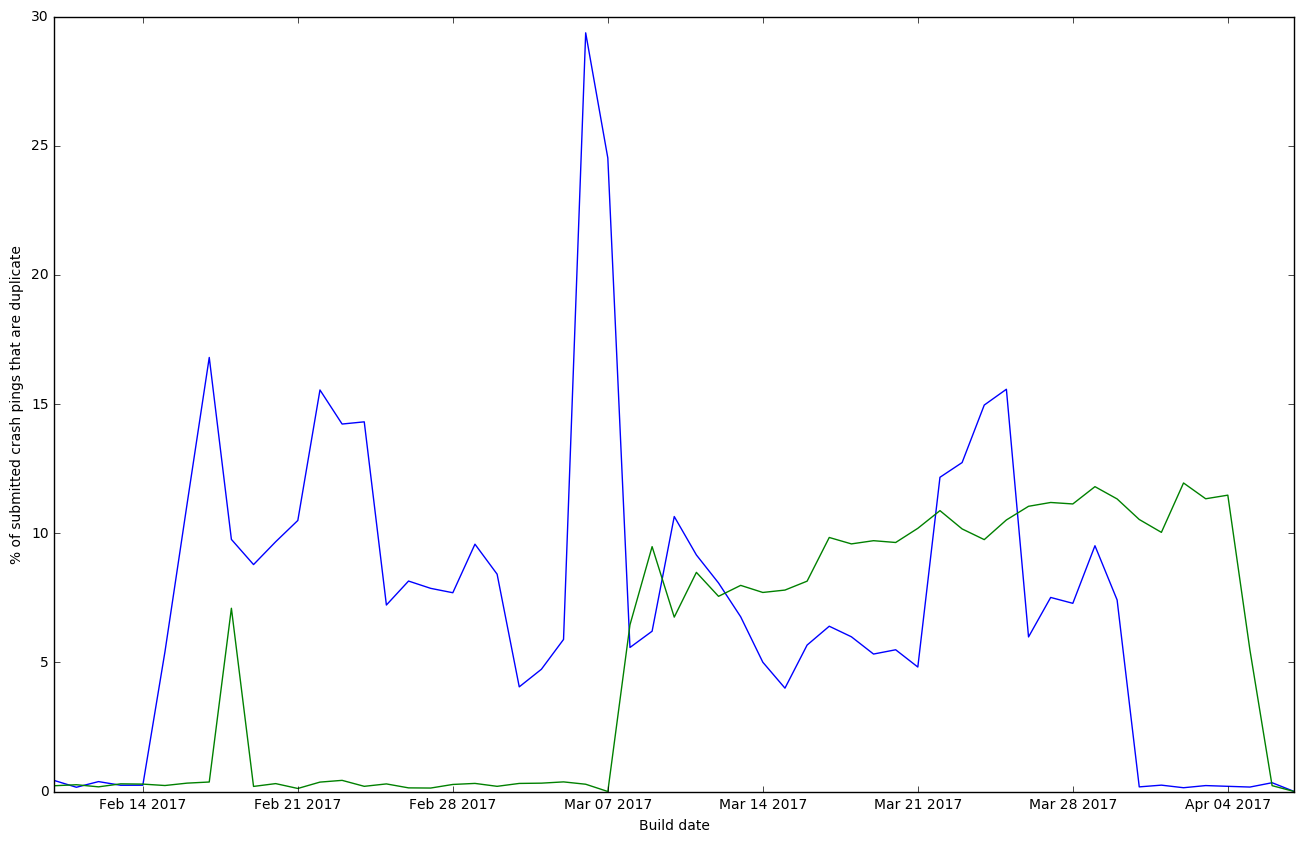### How many duplicate crash pings are we receiving on Nightly/Aurora from 2017-02-10 - 2017-04-07?

import pandas as pd
import numpy as np
import matplotlib

from matplotlib import pyplot as plt
from moztelemetry.dataset import Dataset
from moztelemetry import get_pings_properties, get_one_ping_per_client

Unable to parse whitelist (/mnt/anaconda2/lib/python2.7/site-packages/moztelemetry/histogram-whitelists.json). Assuming all histograms are acceptable.

pings = Dataset.from_source("telemetry")\
.where(docType='crash')\
.where(appName='Firefox')\
.where(appUpdateChannel=lambda x: x == 'nightly' or x == 'aurora')\
.where(appBuildId=lambda x: x > '20170210' and x < '20170408')\
.records(sc, sample=1)

subset = get_pings_properties(pings, ["id", "application/channel", "application/buildId"])


To get the proportions of each builds’ crash pings that were duplicated, get the full count and the deduplicated count per-build.

build_counts = subset.map(lambda s: ((s["application/buildId"][:8], s["application/channel"]), 1)).countByKey()

deduped_counts = subset\
.map(lambda s: (s["id"], s))\
.reduceByKey(lambda a, b: a)\
.map(lambda pair: pair)\
.map(lambda s: ((s["application/buildId"][:8], s["application/channel"]), 1)).countByKey()

from datetime import datetime

sorted_counts = sorted(build_counts.iteritems())

sorted_deduped = sorted(deduped_counts.iteritems())

plt.figure(figsize=(16, 10))
plt.plot([datetime.strptime(k, '%Y%m%d') for k,v in sorted_deduped if k == 'nightly'], [100.0 * (build_counts[k] - v) / build_counts[k] for k,v in sorted_deduped if k == 'nightly'])
plt.plot([datetime.strptime(k, '%Y%m%d') for k,v in sorted_deduped if k == 'aurora'], [100.0 * (build_counts[k] - v) / build_counts[k] for k,v in sorted_deduped if k == 'aurora'])
plt.ylabel("% of submitted crash pings that are duplicate")
plt.xlabel("Build date")
plt.show()#### Conclusion:

Looks like something happened on March 30 on Nightly and April 5 on Aurora to drastically reduce the proportion of duplicate crash pings we’ve been seeing.